Planar, Plane and Non-Planar Graphs

Prepared by:

Joseph Malkevitch
Department of Mathematics
York College (CUNY)
Jamaica, New York 11451

email:

malkevitch@york.cuny.edu

web page:

http://york.cuny.edu/~malk/

If you want to sketch a diagram or write something down, it is helpful to have a piece of paper. People often carry around or have easily available paper, while spheres and tori are harder to come by. Thus, it is of special interest to know the properties of diagrams, such as those of graphs, which can be represented "nicely" on a flat piece of paper (or blackboard).

The diagram (graph G) shown in Figure 1 has 4 vertices and 4 edges, and the point where the two edges cross but which is not a vertex can be eliminated by drawing an isomorphic version of the graph shown.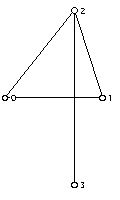Figure 1

Two graphs isomorphic to G are shown in Figure 2.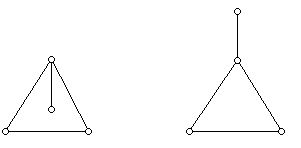Figure 2

The advantage that either of these drawings has over the drawing in Figure 1 is that edges meet only at vertices.

A graph which is isomorphic to a graph which can be drawn in the plane so that its edges only meet at vertices is known as a planar graph. A graph which has been drawn in the plane so that its edges meet only at vertices is known as a plane graph and is said to have been embedded in the plane. The graph in Figure 1 is planar but not plane while both graphs shown in Figure 2 are plane (as well as being planar).

The graph Q displayed in Figure 3 shows the way that a "rectangular solid" is often displayed so as to suggest its 3-dimensional character.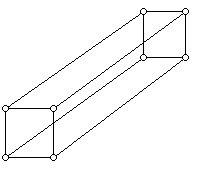Figure 3

However, the drawing of Q in Figure 4 shows that Q is a planar graph, by displaying a plane graph isomorphic to Q. In fact, the graphs of all convex 3-dimensional polyhedra are planar.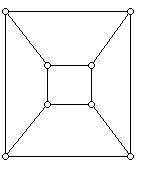Figure 4

Graphs which are plane have not only vertices and edges, but also "faces." Intuitively, faces are regions in the graph drawing where to get between any two points of the region one can do so using a "curve/segment" which crosses no edges of the graph. However, in every plane graph there is one region which is unbounded. This face is often referred to as the infinite face or unbounded face. All of the other faces of a graph are bounded faces, in the sense that one can draw a circle which will enclose one of such faces completely. Just as one can count the number of edges at a vertex of a (simple) graph, one can count the number of sides that each of the regions which make up the faces of a plane graph has.

It is common to denote the number of faces of a plane graph with k sides by pk. Just as for graphs which allow loops it is convenient to say that a loop contributes 2 to the valence of the vertex it is present at, if a plane graph has an edge whose removal will disconnect the graph, it is convenient to have this edge contribute 2 to the number of sides of the face. Thus, the infinite face for the graph on the right in Figure 2 has 5 sides. The other face of this graph has 3 sides. For the graph on the left in Figure 2, the infinite face has 3 sides and the bounded face has 5 sides. All of the faces for the graph in Figure 4 are 4-gons, so for this graph we have p4 is 6.

Notice that just as when one sums the valences of the vertices of any graph one counts all of the edges twice, when one sums the number of sides of the faces of a plane graph, one counts all of the edges twice. As a corollary of this observation the number of faces in a plane graph with an odd number of sides is even. If one records the number of faces with k sides in a plane graph in increasing order, one gets what is sometimes referred to as the face vector of the graph. For example, consider the graph in Figure 5.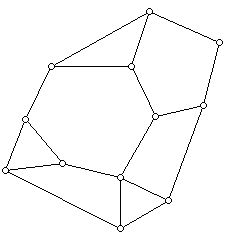Figure 5

For this graph we have p3=3, p4=2, p5=1, p6=1 and p8=1. Here the infinite face has 8 sides. Note that the face vector of a plane graph is the analogue for faces of the graph what the degree sequence is for the vertices of the graph. In fact, using the idea of "duality" one can see how these two sequences are related to each other for plane graphs. The situation here is rather subtle because it is possible for two isomorphic plane graphs to have different face vectors. Can you find an example to show this?

We have seen that some graphs which have crossings at points other than vertices when drawn in the plane can be redrawn so as to eliminate the crossings. Are there graphs which can never be represented in the plane without crossings at points other than the vertices of the graph?

It turns out there are infinitely many such graphs. Two examples are shown in Figure 6 and Figure 7.Figure 6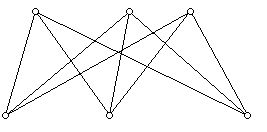Figure 7

Figure 8 shows a graph isomorphic to the one in Figure 6. This graph is known as the complete graph on 5 vertices because every vertex is joined to every other vertex by an edge.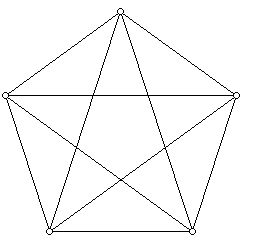Figure 8

Note that the drawing in Figure 6 has fewer points where edges meet which are not vertices than the drawing in Figure 8. This is related to the idea of finding the crossing number for a graph on a particular surface.

Somewhat surprisingly, it turns out that every graph which cannot be drawn in the plane so that its edges meet only at vertices, must "contain" a copy of either the graph in Figure 7 or Figure 8. A graph which cannot be drawn in the plane so that its edges meet only at vertices is called non-planar. Non-planar graphs were characterized using purely graph theory ideas by the Polish mathematician K. Kuratowski. The characterization makes precise in what sense every non-planar graph "contains a copy" of either the graph in Figure 7 or Figure 8. In honor of Kuratowski, the graph in Figure 8 is denoted K5, the complete graph on 5 vertices, while the graph in Figure 7 is denoted K3,3. This graph is one in the family of complete bipartite graphs. A graph is bipartite if the vertices in the graph can be placed into two sets X and Y so that any edges of the graph only go between the sets X and Y. There are no edges between the vertices in X or in Y. It is easy to see that a graph is bipartite if and only if all its circuits have even length.

The Jordan Curve Theorem and Euler's Polyhedral Formula are essential tools in investigating this circle of ideas. Major questions concern being able to tell whether a particular graph is planar or not. What is the complexity of algorithms which determine planarity? If a graph is non-planar what is the smallest number of crossings with which it can be drawn in the plane? Also, many interesting classes of graphs are planar, including the graphs which are the vertex-edge graphs of polyhedra that are homeomorphic to a sphere. Other interesting questions concern symmetrical drawings of plane graphs in the plane. If one gets tired of plane graphs, one can ask similar questions for other surfaces such as the projective plane or torus.

Back to list of tidbits